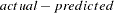## Variance Model in PROC MODEL

Hi,
I am trying to implement various GARCH models using the PROC model statement, but having problems.

I am comparing the results from some examples provided in SAS Support:

rsubmit;

%let df = 7.5;
%let sig1 = 1;
%let sig2 = 0.1 ;
%let var2 = 2.5;
%let nobs = 1000 ;
%let nobs2 = 2000 ;
%let arch0 = 0.1 ;
%let arch1 = 0.2 ;
%let garch1 = 0.75 ;
%let intercept = 0.5 ;

data normal;
lu = &var2;
lh = &var2;
do i= -500 to &nobs ;
/* GARCH(1,1) with normally distributed residuals */
h = &arch0 + &arch1*lu**2 + &garch1*lh;
u = sqrt(h) * rannor(12345) ;
y = &intercept + u;
lu = u;
lh = h;
if i > 0 then output;
end;
run;

proc autoreg data = normal ;
/* Estimate GARCH(1,1) with normally distributed residuals with AUTOREG*/
model y = / garch = ( q=1,p=1 ) ;
output out = normal p = pred r = resid cev = vhat;

run ;
quit ;

/* Estimate GARCH(1,1) with normally distributed residuals with MODEL*/
proc model data = normal ;
/* mean model */
y = intercept ;
/* variance model */
h.y = arch0 + arch1*xlag(resid.y**2,mse.y) +
garch1*xlag(h.y,mse.y) ;
/* fit the model */
fit y / method = marquardt outall outest = ests out = NORMALB fiml ; ;
run ;
quit ;
endrsubmit;

When I look at the Residuals in NORMALB, they do not appear to make sense. So, I was expecting RESIDUAL to equal PREDICTED - EXPECTED = 0.479 - -1.035 = 1.51 vs the output in NORMALB = -0.864. Where does this value come from?

Also, the PROC AUTOREG model has a nice output parameter cev = zzz which outputs the volatility estimate - is there an equivalend method of extracting volatility estimates from the PROC MODEL h.zzz model for variance.

I need to use the PROC MODEL approach rather than PROC AUTOREG as PROC MODEL allows more exotic GARCH models than PROC AUTOREG.

Hope somebody can help.
Thanks!
2 REPLIES 2

## Re: Variance Model in PROC MODEL

Hey,

I would like to use the proc model for estimating a garch model, but I don't know how to extract the volatility estimated.

DO you find the solution to extract the volatility with this procedure?

Thanks a lot,

Anaïs

## Re: Variance Model in PROC MODEL

As for the cev, I think there is an easy way to get. Under PROC MODEL, in FIT statement, check "OUTRESID":

"...If the h.var equation is specified, the residual values written to the OUT= data set are the normalized residuals, defined as, divided by the square root of the h.var value..."

note that H.name variable specifies the functional form for the variance of the named equation

That means that you can derive h.var (cev) by using actual, predicted, and residual outputs.